# Pile Foundation_Neeraj_RITES.pptx

1 Jun 2023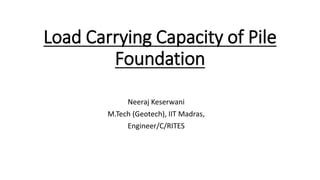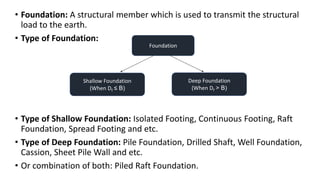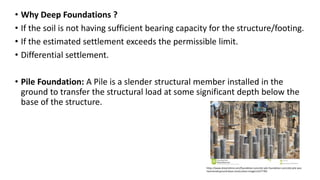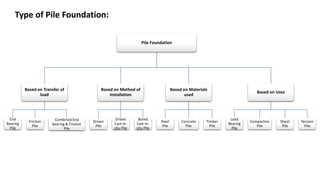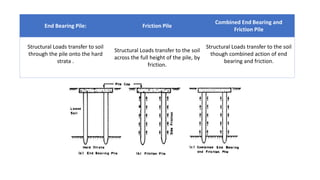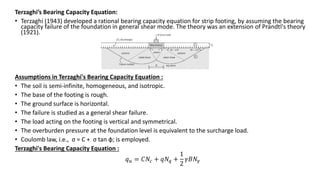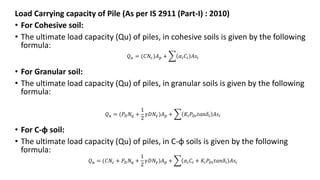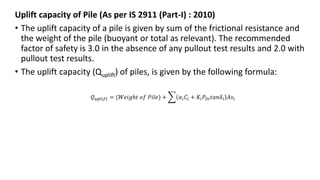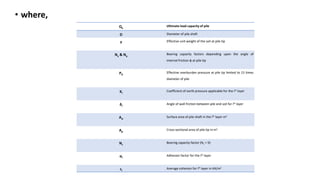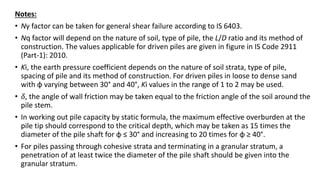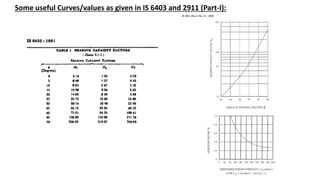1 sur 12

### Pile Foundation_Neeraj_RITES.pptx

• 1. Load Carrying Capacity of Pile Foundation Neeraj Keserwani M.Tech (Geotech), IIT Madras, Engineer/C/RITES
• 2. • Foundation: A structural member which is used to transmit the structural load to the earth. • Type of Foundation: • Type of Shallow Foundation: Isolated Footing, Continuous Footing, Raft Foundation, Spread Footing and etc. • Type of Deep Foundation: Pile Foundation, Drilled Shaft, Well Foundation, Cassion, Sheet Pile Wall and etc. • Or combination of both: Piled Raft Foundation. Foundation Shallow Foundation (When Df ≤ B) Deep Foundation (When Df > B)
• 3. • Why Deep Foundations ? • If the soil is not having sufficient bearing capacity for the structure/footing. • If the estimated settlement exceeds the permissible limit. • Differential settlement. • Pile Foundation: A Pile is a slender structural member installed in the ground to transfer the structural load at some significant depth below the base of the structure. https://www.dreamstime.com/foundation-concrete-pile-foundation-concrete-pile-was- hammered-ground-base-construction-image114377783
• 4. Type of Pile Foundation: Pile Foundation Based on Transfer of load End Bearing Pile Friction Pile Combined End bearing & Friction Pile Based on Method of installation Driven Pile Driven Cast-in- situ Pile Bored Cast-in- situ Pile Based on Materials used Steel Pile Concrete Pile Timber Pile Based on Uses Load Bearing Pile Compaction Pile Sheet Pile Tension Pile
• 5. End Bearing Pile: Friction Pile Combined End Bearing and Friction Pile Structural Loads transfer to soil through the pile onto the hard strata . Structural Loads transfer to the soil across the full height of the pile, by friction. Structural Loads transfer to the soil though combined action of end bearing and friction.
• 6. Terzaghi’s Bearing Capacity Equation: • Terzaghi (1943) developed a rational bearing capacity equation for strip footing, by assuming the bearing capacity failure of the foundation in general shear mode. The theory was an extension of Prandtl's theory (1921). Assumptions in Terzaghi's Bearing Capacity Equation : • The soil is semi-infinite, homogeneous, and isotropic. • The base of the footing is rough. • The ground surface is horizontal. • The failure is studied as a general shear failure. • The load acting on the footing is vertical and symmetrical. • The overburden pressure at the foundation level is equivalent to the surcharge load. • Coulomb law, i.e., σ = C + σ tan ф; is employed. Terzaghi's Bearing Capacity Equation : 𝑞𝑢 = 𝐶𝑁𝑐 + 𝑞𝑁𝑞 + 1 2 𝛾𝐵𝑁𝛾
• 7. Load Carrying capacity of Pile (As per IS 2911 (Part-I) : 2010) • For Cohesive soil: • The ultimate load capacity (Qu) of piles, in cohesive soils is given by the following formula: 𝑄𝑢 = (𝐶𝑁𝑐)𝐴𝑝 + 𝛼𝑖𝐶𝑖 𝐴𝑠𝑖 • For Granular soil: • The ultimate load capacity (Qu) of piles, in granular soils is given by the following formula: 𝑄𝑢 = (𝑃𝐷𝑁𝑞 + 1 2 𝛾𝐷𝑁𝛾)𝐴𝑝 + 𝐾𝑖𝑃𝐷𝑖𝑡𝑎𝑛𝛿𝑖 𝐴𝑠𝑖 • For C-φ soil: • The ultimate load capacity (Qu) of piles, in C-φ soils is given by the following formula: 𝑄𝑢 = (𝐶𝑁𝑐 + 𝑃𝐷𝑁𝑞 + 1 2 𝛾𝐷𝑁𝛾)𝐴𝑝 + 𝛼𝑖𝐶𝑖 + 𝐾𝑖𝑃𝐷𝑖𝑡𝑎𝑛𝛿𝑖 𝐴𝑠𝑖
• 8. Uplift capacity of Pile (As per IS 2911 (Part-I) : 2010) • The uplift capacity of a pile is given by sum of the frictional resistance and the weight of the pile (buoyant or total as relevant). The recommended factor of safety is 3.0 in the absence of any pullout test results and 2.0 with pullout test results. • The uplift capacity (Quplift) of piles, is given by the following formula: 𝑄𝑢𝑝𝑙𝑖𝑓𝑡 = (𝑊𝑒𝑖𝑔ℎ𝑡 𝑜𝑓 𝑃𝑖𝑙𝑒) + 𝛼𝑖𝐶𝑖 + 𝐾𝑖𝑃𝐷𝑖𝑡𝑎𝑛𝛿𝑖 𝐴𝑠𝑖
• 9. • where, Qu Ultimate load capacity of pile D Diameter of pile shaft γ Effective unit weight of the soil at pile tip Nγ & Nq Bearing capacity factors depending upon the angle of internal friction φ at pile tip PD Effective overburden pressure at pile tip limited to 15 times diameter of pile Ki Coefficient of earth pressure applicable for the ith layer δi Angle of wall friction between pile and soil for ith layer Asi Surface area of pile shaft in the ith layer m2 Ap Cross-sectional area of pile tip in m2 Nc Bearing capacity factor (Nc = 9) αi Adhesion factor for the ith layer ci Average cohesion for ith layer in kN/m2
• 10. Notes: • Nγ factor can be taken for general shear failure according to IS 6403. • Nq factor will depend on the nature of soil, type of pile, the L/D ratio and its method of construction. The values applicable for driven piles are given in figure in IS Code 2911 (Part-1): 2010. • Ki, the earth pressure coefficient depends on the nature of soil strata, type of pile, spacing of pile and its method of construction. For driven piles in loose to dense sand with φ varying between 30° and 40°, Ki values in the range of 1 to 2 may be used. • 𝛿, the angle of wall friction may be taken equal to the friction angle of the soil around the pile stem. • In working out pile capacity by static formula, the maximum effective overburden at the pile tip should correspond to the critical depth, which may be taken as 15 times the diameter of the pile shaft for φ ≤ 30° and increasing to 20 times for φ ≥ 40°. • For piles passing through cohesive strata and terminating in a granular stratum, a penetration of at least twice the diameter of the pile shaft should be given into the granular stratum.
• 11. Some useful Curves/values as given in IS 6403 and 2911 (Part-I):